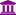# "Pivot operator"

Member, University Professor Posts: 10University Professor
edited June 2019 in Help
Hello. We are trying to use the pivot operator.

We want to collapse the rows that have the same date with summing of the values of the variables.

So that a table like this
Date Variable1 Variable2 Variable3 Variable4
2012/04/20 5 2 2 6
2012/04/20 6 5 2 6
2012/04/20 5 8 6 2
2012/04/21 5 7 2 2
2012/04/21 8 6 2 6
2012/04/22 8 4 8 9
2012/04/22 5 5 5 8

Would become this:
Row Labels Sum of Variable1 Sum of Variable2 Sum of Variable3 Sum of Variable4
4/20/2012 16 15 10 14
4/21/2012 13 13 4 8
4/22/2012 13 9 13 17
Grand Total 42 37 27 39

Can anyone suggest what to do to get this?
Thanks!
Tagged:

• RapidMiner Certified Expert, Member Posts: 458Unicorn
`<?xml version="1.0" encoding="UTF-8" standalone="no"?><process version="5.2.006">  <context>    <input/>    <output/>    <macros/>  </context>  <operator activated="true" class="process" compatibility="5.2.006" expanded="true" name="Process">    <process expanded="true" height="206" width="748">      <operator activated="true" class="generate_sales_data" compatibility="5.2.006" expanded="true" height="60" name="Generate Sales Data" width="90" x="112" y="120">        <parameter key="number_examples" value="100000"/>      </operator>      <operator activated="true" class="select_attributes" compatibility="5.2.006" expanded="true" height="76" name="Select Attributes" width="90" x="246" y="120">        <parameter key="attribute_filter_type" value="subset"/>        <parameter key="attributes" value="|single_price|date|amount"/>        <parameter key="include_special_attributes" value="true"/>      </operator>      <operator activated="true" class="date_to_nominal" compatibility="5.2.006" expanded="true" height="76" name="Date to Nominal" width="90" x="380" y="120">        <description>Sun Apr 01 09:09:06 BST 2007</description>        <parameter key="attribute_name" value="date"/>        <parameter key="date_format" value="yyyyMMdd"/>      </operator>      <operator activated="true" class="aggregate" compatibility="5.2.006" expanded="true" height="76" name="Aggregate" width="90" x="514" y="120">        <list key="aggregation_attributes">          <parameter key="single_price" value="average"/>          <parameter key="amount" value="sum"/>          <parameter key="single_price" value="count"/>        </list>        <parameter key="group_by_attributes" value="|date"/>        <parameter key="count_all_combinations" value="true"/>        <parameter key="ignore_missings" value="false"/>      </operator>      <operator activated="true" class="sort" compatibility="5.2.006" expanded="true" height="76" name="Sort" width="90" x="688" y="118">        <parameter key="attribute_name" value="date"/>      </operator>      <connect from_op="Generate Sales Data" from_port="output" to_op="Select Attributes" to_port="example set input"/>      <connect from_op="Select Attributes" from_port="example set output" to_op="Date to Nominal" to_port="example set input"/>      <connect from_op="Date to Nominal" from_port="example set output" to_op="Aggregate" to_port="example set input"/>      <connect from_op="Aggregate" from_port="example set output" to_op="Sort" to_port="example set input"/>      <connect from_op="Sort" from_port="example set output" to_port="result 1"/>      <portSpacing port="source_input 1" spacing="0"/>      <portSpacing port="sink_result 1" spacing="0"/>      <portSpacing port="sink_result 2" spacing="0"/>    </process>  </operator></process>`